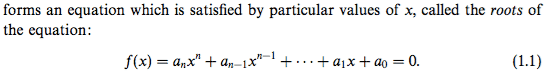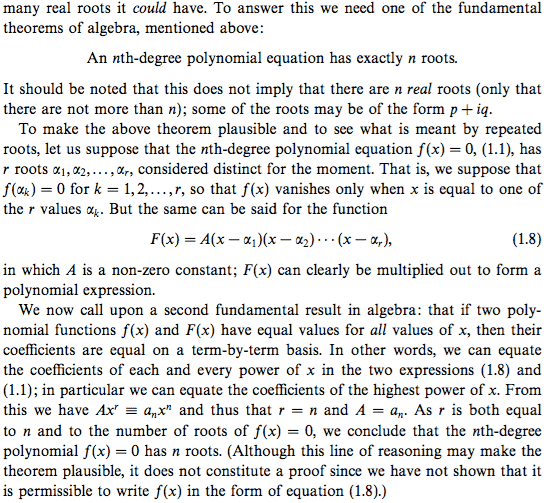# Prove an nth-degree polynomial has exactly n roots

• I
• Happiness

#### Happiness

The attachment below proves that an nth-degree polynomial has exactly ##n## roots.

The outline of the proof is as follows:
Suppose (1.1) has ##r## roots. Then it can be written in the form of (1.8) by factor theorem.
Next use the second fundamental result in algebra (SFRA): if ##f(x)=F(x)## for all values of ##x##, then their coefficients ##a_i##'s in (1.1) are the same. So we have [after expanding (1.8)] ##Ax^r=a_nx^n##. Thus ##r=n##.

The last sentence suggests that if ##f(x)## can be written in the form (1.8) then the proof is complete. And it can be by factor theorem.

My issue with the proof is that the condition for SFRA is not satisfied and hence we cannot compare the coefficients ##a_i##'s. We do not know that ##f(x)=F(x)## for all values of ##x##; we only know ##f(x)=F(x)## for ##r## values of ##x##, for those ##r## roots ##\alpha_i##'s.

So how could we justify the use of SFRA?Last edited:
The last sentence suggests that if ##f(x)## can be written in the form (1.8) then the proof is complete. And it can be by factor theorem.
If you have established that ##f(x)## can be written in the form (1.8), then doesn't that mean precisely that ##f(x) = F(x)## for all ##x##? If not, then what does it mean?

If you have established that ##f(x)## can be written in the form (1.8), then doesn't that mean precisely that ##f(x) = F(x)## for all ##x##? If not, then what does it mean?

Oh, this is a good point!

Then I've not established that ##f(x)## can be written in the form (1.8) with ##A## being a constant, but only that ##f(x)=g(x)(x-\alpha_1)(x-\alpha_2)...(x-\alpha_r)## where ##g(x)## is a polynomial.

Wikipedia: "In spite of its name, there is no purely algebraic proof of the theorem, since any proof must use the completeness of the reals (or some other equivalent formulation of completeness), which is not an algebraic concept." https://en.wikipedia.org/wiki/Fundamental_theorem_of_algebra

Does it mean there is no elementary proof for this theorem?

Last edited:
Wikipedia: "In spite of its name, there is no purely algebraic proof of the theorem, since any proof must use the completeness of the reals (or some other equivalent formulation of completeness), which is not an algebraic concept." https://en.wikipedia.org/wiki/Fundamental_theorem_of_algebra

Does it mean there is no elementary proof for this theorem?
The fundamental theorem of algebra has no really elementary proof. It says that any nonconstant polynomial (with real or complex coefficients) has at least one complex root. So it is really a statement involving complex analysis, and indeed the most straightforward proof uses complex analysis. However, there are many other ways to prove it. Here is a book which proves the theorem six different ways and is intended as a semester-long course at the senior undergraduate level: https://www.amazon.com/dp/0387946578/?tag=pfamazon01-20 by Benjamin Fine and Gerhard Rosenberger. So that gives you some idea of the level of mathematics required for a rigorous proof.

However, note that if we assume the fundamental theorem of algebra, then it is not hard to show that your ##f(x)## can be written in the form ##A(x-x_1)(x-x_2)\cdots(x-x_n)##. We can do this by induction on ##n##, the degree of ##f(x)##. First, it is clear that if ##n=0## or ##n=1## then ##f(x)## is already in that form. So suppose that ##n \geq 2## and that the result is true for all polynomials of degree smaller than ##n##, and that ##f(x)## has degree ##n##. By the fundamental theorem of algebra, ##f(x)## has at least one complex root, call it ##x_1##. By the division algorithm, we may write ##f(x) = g(x)(x-x_1) + r(x)##, where ##g(x)## is a polynomial and ##r(x)## is zero or a polynomial of degree less than ##1##, i.e., ##r(x)## is a constant, so just write it as ##r##. Thus ##f(x) = g(x)(x-x_1) + r##. Plugging in ##x=x_1## shows that ##r=0##. Consequently, ##f(x) = g(x)(x-x_1)##. Comparing degrees, we see that ##g(x)## must have degree ##n-1##, so the induction hypothesis applies, and we can write ##g(x) = A(x-x_2)(x-x_3)\cdots(x-x_n)##, and therefore ##f(x) = A(x-x_1)(x-x_2)\cdots(x-x_n)## as desired.

Last edited by a moderator:
•Happiness